cancel
Showing results for
Did you mean:Frequent Visitor

## display all the rows of first column that were filtered by a second column

 Column 1 Column 2 Flag A B 1 B C 1 D A 1 E D 1

I have a table like this. I am able to filter the table by both the columns using a measure. For example, the output table for a filter on both columns 1 and 2 for 'B' looks like this

 Column 1 Column 2 Flag A B 1 B C 1

I want a visual that displays only Column 1 and Flag in a Matrix form. The visual I get at the moment

 Column 1 Flag B 1

However, I want my visual to look like below

 Column 1 Flag A 1 B 1

1 ACCEPTED SOLUTION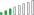Resolver III

Assuming you have a Disconnected Table with values in both Column 1 and Column 2. If you have that already, good, other wise, you can create that like with a New Calculated Table like this:

CombinedValues =
UNION(VALUES('MyTable'[Column1]), VALUES('MyTable'[Column2])). Rename the column created here as "Slice"

Then create a Measure for your flag as below

FlagDisplay =
CALCULATE (
SUM ( 'MyTable'[Flag] ),
KEEPFILTERS (
'MyTable'[Column1]
IN VALUES ( CombinedValues[Slice] )
|| 'MyTable'[Column2] IN VALUES ( CombinedValues[Slice] )
)
)

If this works for you, please mark it as a solution so it can be found easily by anyone who may have similar issues.

10 REPLIES 10Resolver III

Assuming you have a Disconnected Table with values in both Column 1 and Column 2. If you have that already, good, other wise, you can create that like with a New Calculated Table like this:

CombinedValues =
UNION(VALUES('MyTable'[Column1]), VALUES('MyTable'[Column2])). Rename the column created here as "Slice"

Then create a Measure for your flag as below

FlagDisplay =
CALCULATE (
SUM ( 'MyTable'[Flag] ),
KEEPFILTERS (
'MyTable'[Column1]
IN VALUES ( CombinedValues[Slice] )
|| 'MyTable'[Column2] IN VALUES ( CombinedValues[Slice] )
)
)

If this works for you, please mark it as a solution so it can be found easily by anyone who may have similar issues.Frequent Visitor

It works, thank you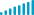Kudo Kingpin

Hi,

In this case I suggest you use a calculated column with the following formula:

Flag = IF('Table'[Column 1] = "B" || 'Table'[Column 2] = "B", 1, BLANK())

After that, add a filter in the visual using this field set to 1.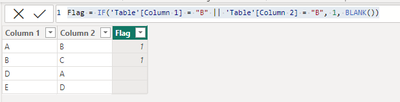Frequent Visitor

that's what I have in the 2nd table. I want a visual table that doesn't include Column 2 but still gives me all rows filtered in Column 1Kudo Kingpin

Yes, please try to follow my suggestion and don't add the second column, it will work 😊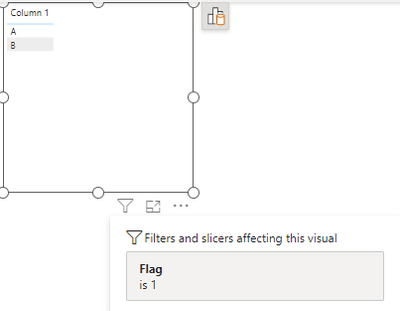Frequent Visitor

yes but this is dynamic, I want it to filter based on a SlicerResolver III

Is "flag" a numeric column?Frequent Visitor

yes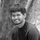Super User

How many slicers do you have ?and what are all the columns you are using?

Thanks,

Arul

If I answered your question then mark my post as a solution and a kudo would be appreciated.Frequent Visitor

just one slicer which has combined values of both column 1 and column 2.